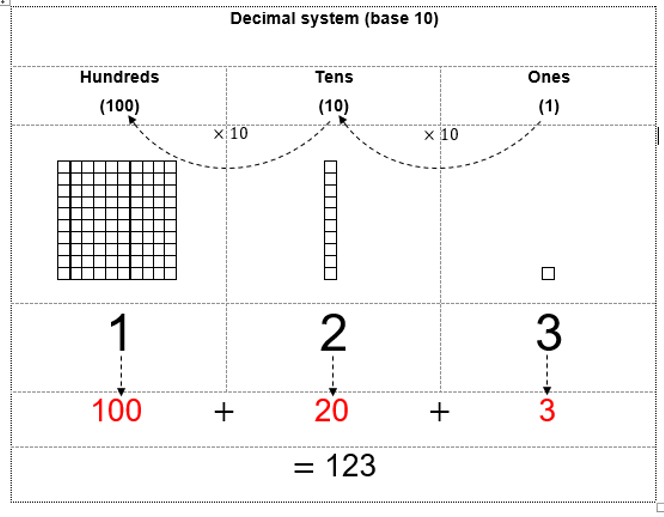Search

# Beautiful Binary Numbers

Trying to nurture an interest in numbers in your children? Why not introduce them to an alternative number system!

The number system that we commonly use is known as the decimal system. The prefix dec- comes from the Greek word deka, which means ten. This is why the decimal system is also known as the base 10 system. The illustration below is a visual representation of the base 10 system.There are other number systems, however. The binary system is one of them, which we will now explore. The prefix bi- comes from Latin, meaning two, which gives the binary system its other name, base 2. In the binary system, each increasing place-value is worth twice of the previous place-value (in the decimal system, the worth of each place-value increases by 10 times). In each place-value, we write either 1 or 0 (in the decimal system, we write 0, 1, 2, 3, 4, 5, 6, 7, 8 or 9).

When you see a letter A on your computer screen, did you know that is actually represented in the computer's brain as a string of numbers made of 1s and 0s. That's right - computers process information in terms of binary numbers. Computers transport, calculate, and translate binary numbers because computer hardware circuits only have two electrical states, on or off. These two states can be represented as zero (off) or one (on). All letters of the alphabet, numbers, and symbols are converted into eight (or more) character binary numbers in digital systems.

However, you can have fun with binary numbers without diving deeply into how computers translate binary numbers into letters, numbers, and characters, which is what we're going to do in the next exercise. Let’s turn our attention to the next illustration, that of the binary system.Count the number of squares above.

How many did you count? In decimal system, we write the number as 42. Conversely, in binary system, we write it as 101010; which is read as one zero one zero one zero. Each of these 1s and 0s are called binary digits, or bits for short.

If your child enjoyed this lesson, they would enjoy Mathematics enrichment at Joyous Learning. Besides teaching children what they need to know for school, we regularly nourish our students with interesting mathematical concepts such as this under the section we call Mad Maths!

Other Mad Maths lessons in our regular Primary School Level Mathematics syllabus include Pythagoras Theorem, Egyptian Fractions, Dollar-Cost-Averaging, the Trachtenberg System of Mental Calculation and more. Click the links on related posts to read more.